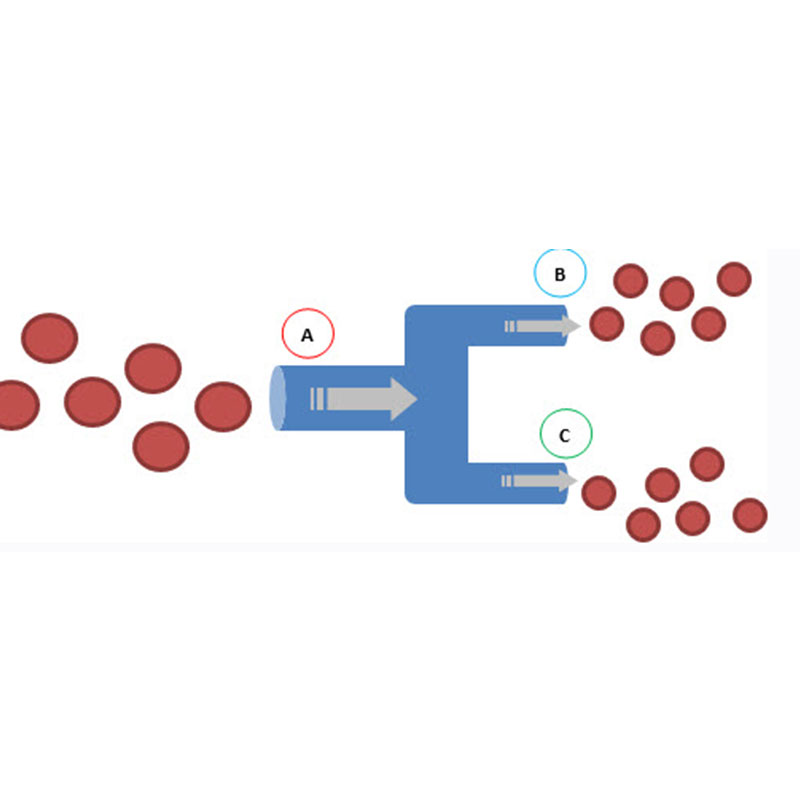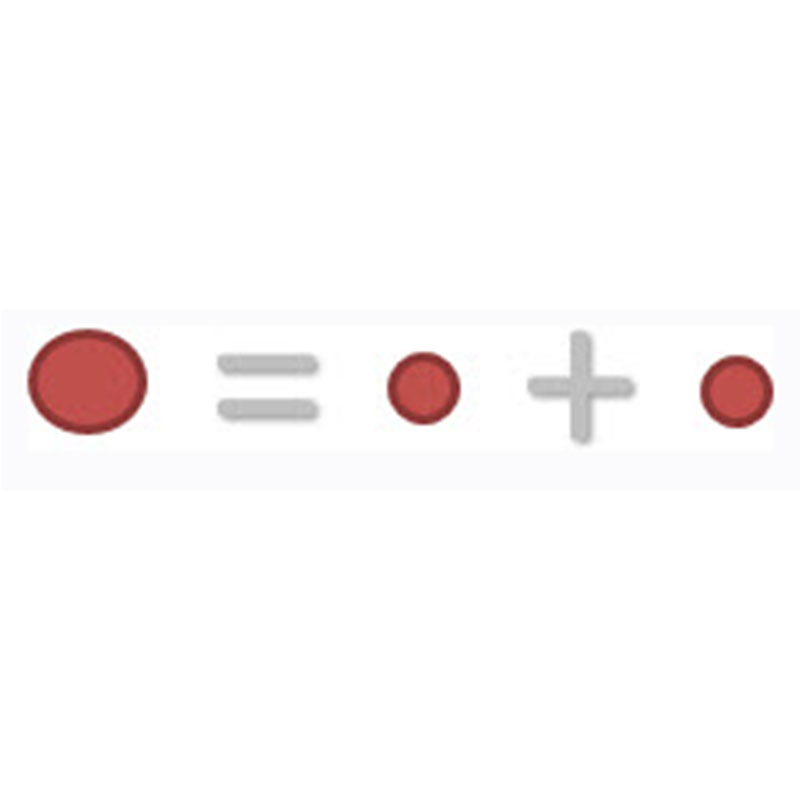Present in a simple way:RF Splitter 2020/03/03

RF Power Divider,as the name implies, divides.

In nature we can see an example of splitter on a river that has an obstacle, and splits into two. In this case, part of the water continues down a path, and another part by another way.In the case of RF 2way Dividers, instead of water, it is the RF signal that is divided - in that case the input signal is 'divided': the form remains unchanged, but the 'power' is splitted. For this reason, the RF dividers are known as RF Power Splitters.

In the following figure, we see a simple illustration of a Splitter. The signal (represented by large red circles) goes in one side and out the other two (B) and (C).Note that the output signal is the same (has the same form), but each output has 'half' the power of the original signal (small red circles).Basically, that's what the divider does.

Categories
Recent Posts
Tags

Do you have any questions ?

Call Us : +86 551 65329702
Subscribe
Please read on, stay posted, subscribe, and we welcome you to tell us what you think.
welcome to maniron